Isoperimetric Sliced PolyformsThese sets could equally well be referred to as sliced isoperimetric polyforms since slicing an isometric set will produce a set of isoperimetric pieces. The solutions below are grouped by the isoperimetric set from which they come, viz by perimeter. At present only the polyomino sets are considered here.

Isoperimetric Sliced Polyominoes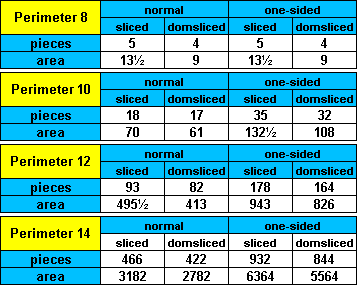Perimeter 8

Sliced, domsliced and sliced + domslicedSliced (one-sided set)Plus a half squarePlus a half square (one sided set)Plus a half domino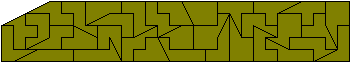Perimeter 10

SlicedSliced (one-sided set)Domsliced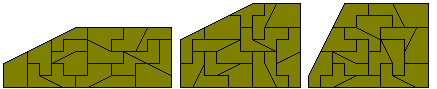Domsliced-domsliced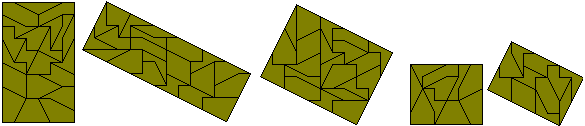Domsliced-domsliced (one-sided set)Plus a half squarePlus a half square (one-sided set)Domsliced and sliced (combined sets)

No rectangle is possible but a number of sliced rectangles can be made.Minus three half squares

One rectangle is possible plus a number of symmetrical patterns found by Roel Huismann.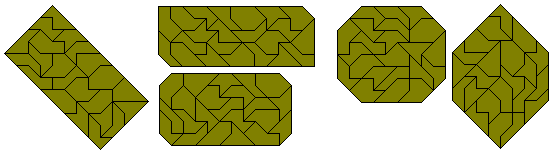Domsliced domsliced

Three rectangles are possible plus a number of symmetrical patterns found by Roel Huismann.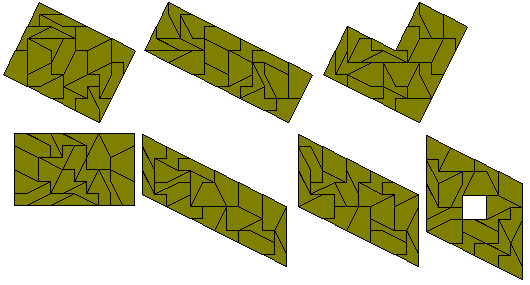Perimeter 12

Sliced

No rectangle is possible with this set unless a piece is used twice as below.A number of sliced rectangles are possible with the full set.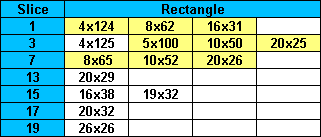The one-sided set consists of 178 pieces covering an area of 943 squares. The only possible rectangle is the 23x41 shown here.Sets of squares can also be made with the full set.A number of sliced rectangles can also be made.Domsliced

The only rectangle possible with this set is the 7x59 below together with some multiple square constructions. The maximum possible number of different sized squares is four with two possibilities - 6, 8, 12, 13 and 6, 9, 10, 14. For three squares we could have 4, 6, 19; 5, 8, 18; 6, 11, 16 or 10, 12, 13.Sliced rectangles are possible as shown in the table below. The 3x139 cannot be formed owing to a lack of suffient straight edging from the pieces. For some of the remainder there are two solutions for each rectangle depending on the direction of the sliceThe one-sided set consists of 164 pieces covering an area of 826 squares. They are shown here in two 7x 59 rectangles.A number of sliced rectangles can also be made.Perimeter 14

There are 466 sliced perimeter 14 polyominoes with area 3182 which will probably form two 37x43 rectangles.and 422 domsliced perimeter 14 polyominoes with area 2782 which could well make two 13x107 rectangles.

The fixed area/fixed perimeter sets may be easier to find solution for.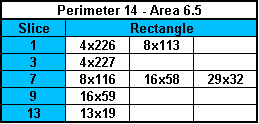Isoperimetric Sliced Polyiamonds

Perimeter 7